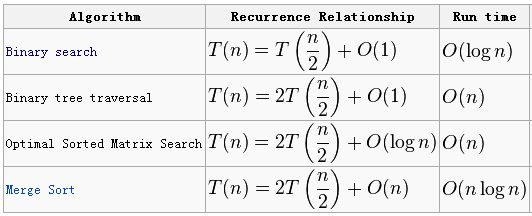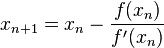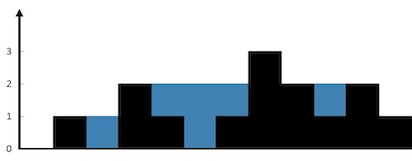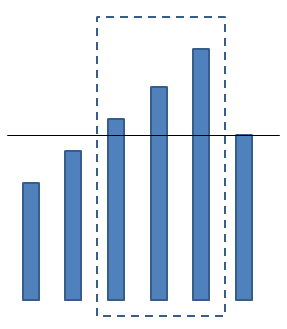（下篇）
LeetCode

Quan Wang <quanrpi@gmail.com>
2016.01.06

LeetCode使用方法
Internal Error: 去掉print的语句
Output Limit Exceeded: 返回的集合的长度大于正确答案
Time Limit Exceeded: 用时过长，需要优化

1. hash table （HashMap，HashSet）
2. recursion
3. dynamic programming
4. backtracking回溯法（8 queens, parentheses, permutations, combinations, combination sum）
5. bit, XOR, a^b^b=a
6. array的修改或合并，从后往前填充元素
7. 创建新array，从后往前构建，记录总和、最大值等
8. 不能用recursion：用stack
9. String太慢，用StringBuffer，方法有append(String s), insert(int offset, String s), toString()
10. 排序后用头尾指针(K-Sum, container with most water)
11. 连续最大和：running sum（书上的17.8以及18.12）
12. substring，用suffix tree
13. 找最大的，就从最大的开始试，然后试小的
14. 树的常用思路：将节点的取值范围作为参数进行递归（例如判断BT是否是BST）；在节点记录子树的大小；全局变量记录上次访问的节点
15. anagram：character count，sort string。回文：中间往两边搜。

1. 当前是第几步
2. 当前结果
3. 用来放最终结果的集合
rec函数中关键步骤：
2. 否则，向前一步尝试新的当前结果
3. 递归调用rec，使用新的结果
4. 把当前结果再改回来

Main Recurrence Theorem (Master Theorem)
T(n)=aT(n/b)+f(n)
f(n)=O(n^k)Single Number，Single Number II以及Single Number III

for(int i=0;i<A.length;i++)
{
int newone=one|(three&A[i]);
int newtwo=two|(one&A[i]);
int newthree=three|(two&A[i]);
one=newone&(~(one&A[i]));
two=newtwo&(~(two&A[i]));
three=newthree&(~(three&A[i]));
}

Divide Two Integers

Trick：从c=1开始对divisor进行位运算，divisor每乘以2，将c也乘以2，直到divisor再乘以2就要大于dividend为止。求差，对差再次进行。

Unique Paths

Set Matrix Zeroes

constant space solution: 用矩阵的第一行和第一列做标记。

Sqrt(x)x_(n+1) = x_n ( 1.5 - a/2 * x_n ^ 2 )

Pow(x, n)

x n = x n/2 * x n/2 * x n%2

Best Time to Buy and Sell Stock

O(n)时间、O(1)空间的算法：记录low和high还有profit。碰到比low更小的就更新low，并清除high。碰到比high更大的就更新high并检查是否更新profit。

Best Time to Buy and Sell Stock II

Best Time to Buy and Sell Stock III（技巧题）

PreProcessing：从左往右扫，记录最小；从右往左扫，记录最大。

Container With Most Water （经典题！）

O(n)的复杂度。保持两个指针i,j；分别指向长度数组的首尾。如果ai 小于aj，则移动i向后（i++）。反之，移动j向前（j--）。如果当前的area大于所记录的area，替换之。这个想法的基础是，如果i的长度小于j，无论如何移动j，短板在i，不可能找到比当前记录的area更大的值了，只能通过移动i来找到新的可能的更大面积。

Trapping Rain Water （经典题！）Two Sum （经典基础题！）

K-sum问题

K>2时，复杂度为O(N^(K-1))。

2Sum的线性解法：

4Sum的O(N^2)解法：

3Sum Smaller

Candy

You are giving candies to these children subjected to the following requirements:
• Each child must have at least one candy.
• Children with a higher rating get more candies than their neighbors.
What is the minimum candies you must give?

Jump Game

O(N)，记录一下每一步时当前能达到的最远距离maxReach即可。

Jump Game II

Largest Rectangle in Histogram（很难！）

(1) O(N^3) approach: for every pair of bars i, j, find its area of the rectangle using i as the left bound and j as the right bound. To calculate the area, one needs to find the shortest bar between i and j, so the total time would be O(N^2) * O(N) which is O(N^3).

(2) O(N^2) approach: one doesn’t have to scan the bars between i and j everytime to find the shortest bar in approach (1). One can always keep a the current shortest bar and each time use const time to update this value. So the time complexity would be reduced as O(N^2).

(3) O(N) approach: this approach is really not easy to come up with. The idea is that, each bar say H defines a maximum rectangle which use this bar H as the shortest histogram in it. H defines this maximum rectangle by finding both the first left and the first right side bar to H which is shorter than H. If we can find the left and right bounds for each bar in linear time, then another linear scan could give us the global maximum rectangle by finding the maximum value over height[i] * (rightbound[i] – leftbound[i] – 1).

Increasing stack is such a data structure helping us to find all the bounds in linear time.  For example, to find the right bound for each bar i (the right bound of i is just the index of the first bar shorter than bar i on the right side of i), we continue to push the histogram into the stack until we get a shorter histogram (we call this shorter histogram as the current histogram) than the one on the top of the stack. Ok, obviously, this shorter one is the right bound of the top histogram. We then keep poping the stack until we get a histogram which is shorter than the current histogram. All the poped out histogram will use the current histogram as their right bound (think about it, this is correct). We repeat this process, until all the histogram are pushed into the stack. Finally, we need to clear the stack, and set the right bound of the remaining histograms in that stack. Since each histogram is just pushed and poped once, this process cost O(N) time. Similarly, we can get the left bounds. Then a third linear scan will give us the global maximum rectangle as previously discussed.Maximal Rectangle

Gas Station（难题）

O(N)的解法：建立两个变量sum和total，从0到length遍历gas[]和cost[]，sum和total都+=gas[i]-cost[i]，检测sum是否小于0，如果是，res变量(restart)等于当前index+1，同时sum归零。最终根据total变量是否大于零决定返回res或者-1。

First Missing Positive（技巧题）

Longest Consecutive Sequence（经典题！）

requirement: O(n)

Median of Two Sorted Arrays （难题）

int findKth(int A[], int B[], int a0, int a1, int b0, int b1, int k)

Maximum Product Subarray

Majority Element

Majority Element II

Rotate Array

9 -> 5 -> 1 -> 7 -> 3 -> (9)
8 -> 4 -> 0 -> 6 -> 2 -> (8)

Find Peak Element

Maximum Gap

Contains Duplicate II

value -> [indices]

Contains Duplicate III

House Robber

Minimum Size Subarray Sum

Product of Array Except Self

Paint House

Same Tree

Unique Binary Search Trees

http://cs.lmu.edu/~ray/notes/binarytrees/
Let C(n) be the number of distinct binary trees with n nodes. This is equal to the number of trees that have a root, a left subtree with j nodes, and a right subtree of (n-1)-j nodes, for each j. That is,

C(n) = C(0)C(n-1) + C(1)C(n-2) + ... + C(n-1)C(0)

Unique Binary Search Trees II

Populating Next Right Pointers in Each Node

Populating Next Right Pointers in Each Node II

Binary Tree Inorder Traversal，Binary Tree Postorder Traversal以及Binary Tree Preorder Traversal

An algorithm by Joseph M. Morris runs in O(n log n) time.
Morris, Joseph M. "Traversing binary trees simply and cheaply." Information Processing Letters 9.5 (1979): 197-200.

Binary Tree Level Order Traversal II

Binary Tree Maximum Path Sum

Recover Binary Search Tree

Construct Binary Tree from Inorder and Postorder Traversal

Construct Binary Tree from Preorder and Inorder Traversal

Count Complete Tree Nodes

Verify Preorder Sequence in Binary Search Tree（难题！）

class Solution(object):
def verifyPreorder(self, preorder):
"""
:type preorder: List[int]
:rtype: bool
"""
inorder = []
stack = []
for p in preorder:
if len(inorder) > 0 and inorder[-1] > p:
return False
while len(stack) > 0 and stack[-1] < p:
inorder.append(stack.pop())
stack.append(p)
return True

Closest Binary Search Tree Value II

Sudoku Solver

Word Search

Subsets以及Subsets II

Subsets就是一个简单的backtracking。此外还可以用二进制来解，1表示选取，0表示不选取。
Subsets II考虑有重复的元素，简单的改进就是先排序，然后考虑每个unique的元素有多少个，例如有M个。以前这个元素要么0次要么1次，现在可以出现0~M次。

Longest Valid Parentheses

1. 如果为"("，直接放。
2. 如果为")"，分情况：
1. 之前为"("，放入一个"2"；
2. 之前为数字，看数字前面一个是否是"("，如果是则加2。
3. 调用一个mergeNum函数，将stack顶层的数字全部合并。

Evaluate Reverse Polish Notation

Simplify Path

Valid Parentheses

Implement Stack using Queues

Longest Substring Without Repeating Characters

Anagrams

Interleaving String

Scramble String

dp[i][j][k] 代表了s1从i开始，s2从j开始，长度为k的两个substring是否为scramble string。

1. 如果两个substring相等的话，则为true。

2. 如果两个substring中间某一个点，左边的substrings为scramble string，同时右边的substrings也为scramble string，则为true。

3. 如果两个substring中间某一个点，s1左边的substring和s2右边的substring为scramble string, 同时s1右边的substring和s2左边的substring也为scramble string，则为true。

Implement strStr()

brute force就能过。
KMP算法更优。

Valid Number

Roman to Integer以及Integer to Roman

Roman to Integer: 把每个字母及其重复变为数字，再比较大小判断是相加还是相减。从右往左看。
Integer to Roman: 一位一位的变，使用对应位的1,5,10。注意10只有make 9的时候才用。注意99是XCIX，不是IC。
makeDigit(int d, String one, String five, String ten)
Symbol Value
I 1
V 5
X 10
L 50
C 100
D 500
M 1,000

Longest Palindromic Substring（经典题！）

D[i,j]表示从i到j是否是回文。

O(N) time and O(N) space

Minimum Window Substring

HashMap: char -> list of indices in S

Palindrome Partitioning II

O(N^2)的方法：
The key to improve your algorithm is to realize that you are computing too many non necessary values in cut[][]. Imagine you have already computed cut[i][j-1] and you now want to compute cut[i][j]. What can happen? That new letter creates palindromes that ends at index j. There could be several of them, and you have to examine if this creates new possible cuts. The formula becomes:

cut[i][j] = Minimum number of cuts for the word s[i]...s[j];
cut[i][j] = 0 if pal[i][j]
cut[i][j] = min_{i < mid < j} { cut[i][mid] + 1 if pal[mid+1][j] } for all 0 ≤ i < j < len

Hey, this is still O(n^3)! Yes, but we are almost there. Note how now we only use the left part cut[i][mid] and never the right part cut[mid+1][j]. Since what we want is cut[len-1], we will never need to compute a value cut[i][j] for i≠0, what that means, is that we only need the first row of cut. This is how we get back to O(n^2).

The final formula:

minCut[i] = Minimum number of cuts for the word s...s[i];
minCut = 0
minCut[i] = { 1                                            --- if pal[i]
{ min_{ 1 ≤ j ≤ i} {minCut[j] + 1 }  --- if pal[j][i]

Shortest Palindrome

checkPalindrome返回-1，则为回文；返回-2，则不是回文（但也不是OOV），下一步end-=1；返回非负数，则为OOV，end设为非负数的前一个数。

Regular Expression Matching

'.' matches any single character.
'*' matches zero or more of the preceding element.

matching要使两个输入s与p完全匹配，而不是部分匹配。

Wildcard Matching（wildcard通配符）

'?' matches any single character.
'*' matches any sequence of characters (including the empty sequence).

1.对于开头不是'*'的p，第一个子串必须从s开始匹配。
2.对于结尾不是'*'的p，最后一个子串必须在s的尾巴上匹配。

Edit Distance

D(i,j)是将str1(0~i-1)变为str2(0~j-1)的距离。

One Edit Distance

Distinct Subsequences

Basic Calculator

Basic Calculator II

Alien Dictionary

Merge k Sorted Lists

Sort List

Reorder List

1）把整个链表划分成2个等长的子链表，如果原链表长度为奇数，那么第一个子链表的长度多1。
2）翻转第二个子链表；
3）将两个子链表合并。

Permutations

backtracking。考虑n!种变换方式，每种变换方式通过两两换位来实现。记得换位后要换回来！

Permutations II

Next Permutation （经典题！）

1. 从后往前找第一个升序，为2,4，标记num[i]=2。此时i后面的子序列为降序。
2. 从后往前找最小的大于num[i]的，为3，标记num[j]=3。
3. 将num[i]与num[j]互换，（1,3,4,2）然后将i后面的子序列变为升序（1,3,2,4）。

Permutation Sequence

Gray Code

Sort Colors

counting sort很简单但是要two-pass。
one-pass我的解法：保持3个index，用于指向0,1,2的末尾，每一步更新。

N-Queens II

Surrounded Regions

trick在于HashSet的hash code是二位坐标一维化col+row*m。

LRU Cache（经典题！）

Max Points on a Line

HashMap<Line,HashSet<Integer>> map=new HashMap<Line,HashSet<Integer>>();

Maximal Square

The Skyline Problem (难题)

Course Schedule